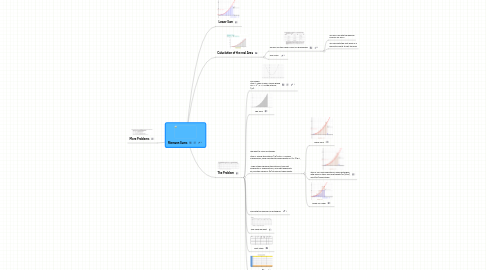# Riemann SumsRogelio Yoyontzin
Comienza Ya. Es Gratis
ó regístrate con tu dirección de correo electrónicoRiemann Sums## 2. Caluclation of the real Area

### 2.1. We will use the Upper Sums ON Blackboard

2.1.1. We will Calcuate the general formula for any n

2.1.2. We calculate the limit when n is going to infinity to get the area

## 3. The Problem

### 3.3. We want to use 8 rectangles Step 2: Divide the interval (1,9) into n = 8 equal subdivisions, each subinterval having width Δx=(9−1)/8=1, Make a table showing the intervals, their left endpoints xL midpoints xM, and right endpoints xR, and the values of f(x) at each of these points

3.3.1. Upper Sum

3.3.2. Step 3: For each subinterval, draw rectangles with base on the x-axis and height f(xL),f(xM), and f(xR) respectively.

3.3.3. Upper vs Lower